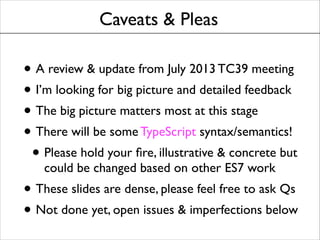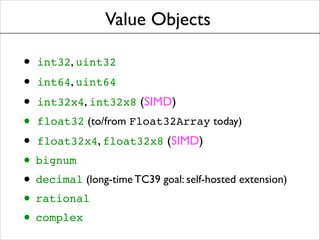Successfully reported this slideshow.

# Value Objects, Full Throttle (to be updated for spring TC39 meetings)×

# Value Objects, Full Throttle (to be updated for spring TC39 meetings)

Slides I prepared for the 29 January 2014 Ecma TC39 meeting, on Value Objects in JS, an ES7 proposal -- this one shotgunned the roadmap-space of declarative syntax, to find the right amount per TC39 (nearly zero, turns out).

Slides I prepared for the 29 January 2014 Ecma TC39 meeting, on Value Objects in JS, an ES7 proposal -- this one shotgunned the roadmap-space of declarative syntax, to find the right amount per TC39 (nearly zero, turns out).

### Value Objects, Full Throttle (to be updated for spring TC39 meetings)

1. 1. Value Objects Brendan Eich brendan@mozilla.com @BrendanEich ! Ecma TC39 January 2014
2. 2. Caveats & Pleas • A review & update from July 2013 TC39 meeting • I’m looking for big picture and detailed feedback • The big picture matters most at this stage • There will be some TypeScript syntax/semantics! • Please hold your ﬁre, illustrative & concrete but could be changed based on other ES7 work • These slides are dense, please feel free to ask Qs • Not done yet, open issues & imperfections below
3. 3. Value Objects • int32, uint32! • int64, uint64! • int32x4, int32x8 (SIMD) • float32 (to/from Float32Array today) • float32x4, float32x8 (SIMD) • bignum • decimal (long-time TC39 goal: self-hosted extension) • rational • complex
4. 4. Overloadable Operators • | ^ &! • ==! • < <=! • << >> >>>! • + -! • * / %! • unary- unary+ boolean-test!! ~
5. 5. Preserving Boolean Algebra • != and ! are not overloadable, to preserve identities including • • • • X ? A : B <=> !X ? B : A !(X && Y) <=> !X || !Y! !(X || Y) <=> !X && !Y X != Y <=> !(X == Y)
6. 6. Preserving Relational Relations • > and >= are derived from < and <= as follows: • • A > B <=> B < A A >= B <=> B <= A • We provide <= in addition to < rather than derive A <= B from !(B < A) in order to allow the <= overloading to match the same value object’s == semantics -- and for special cases, e.g., unordered values (NaNs)
7. 7. Strict Equality Operators • The strict equality operators, === and !==, cannot be overloaded • They work on frozen-by-deﬁnition value objects via a structural recursive strict equality test (beware, NaN !== NaN) • Same-object-reference remains a fast-path optimization
8. 8. Why Not Double Dispatch? • Left-ﬁrst asymmetry (v value, n number): • • v + n ==> v.add(n) n + v ==> v.radd(n)! • Anti-modular: exhaustive other-operand type enumeration required in operator method bodies • Consequent loss of compositionality: complex and rational cannot be composed to make ratplex without modifying source or wrapping in proxies
9. 9. Cacheable Multimethods • Proposed in 2009 by Christian Plesner Hansen (Google) in es-discuss • Avoids double-dispatch drawbacks from last slide: binary operators implemented by 2-ary functions for each pair of types • Supports Polymorphic Inline Cache (PIC) optimizations (Christian was on the V8 team) • Background reading: [Chambers 1992]
10. 10. Binary Operator Example • For v + u with either a value object: ! • Let p = v.[[Get]](@@ADD) • If p is not a Set, throw a TypeError • Let q = u.[[Get]](@@ADD_R) • If q is not a Set, throw a TypeError • Let r = p intersect q • If r.size != 1 throw a TypeError • Let f = r; if f is not a function, throw • Evaluate f(v, u) and return the result
11. 11. API Idea from CPH 2009 // NOTE: NOT PROPOSED FOR ES7 ! function addPointAndNumber(a, b) { return Point(a.x + b, a.y + b); } ! Function.defineOperator('+', addPointAndNumber, Point, Number); ! function addNumberAndPoint(a, b) { return Point(a + b.x, a + b.y); } ! Function.defineOperator('+', addNumberAndPoint, Number, Point); ! function addPoints(a, b) { return Point(a.x + b.x, a.y + b.y); } ! Function.defineOperator('+', addPoints, Point, Point);
12. 12. Literal Syntax • • • • • int64(0) ==> 0L // as in C#! uint64(0) ==> 0UL // as in C#! float32(0) ==> 0f // as in C#! bignum(0) 0n // avoid i/I! ==> decimal(0) ==> 0m // or M, C/F#! • We want a syntax extension mechanism, with declarative not runtime API • This means new syntax for operator function and sufﬁx deﬁnition
13. 13. Value Class Declaration value class point2d { // no suffix // default typeof “point2d” (no overriding/spoofing) ! constructor(private x: int32, private y: int32) { // implicit Object.freeze(this) on return } ! // binary operators (note arrow shorthand for { return … }) point2d + number (a, b) => point2d(a.x + b, a.y); number + point2d (a, b) => point2d(a + b.x, b.y); point2d + point2d (a, b) => point2d(a.x + b.x, a.y + b.y); ! point2d - number (a, b) => point2d(a.x - b, a.y); number - point2d (a, b) => point2d(a - b.x, b.y); point2d - point2d (a, b) => point2d(a.x - b.x, a.y - b.y); ! // more operators with private access elided }
14. 14. Value Class Declaration, cont. value class pixel { // CSS unit, 1/96th of an inch suffix “px”; typeof “CSS:pixel”; // we allow a lot, but overriding throws ! constructor pixel(public twips: int32) { // implicit Object.freeze(this) on return } ! // unary operators (note arrow shorthand for { return … }) +() => pixel(this.twips); -() => pixel(-this.twips); !!() => !!this.twips; ~() => pixel(~this.twips); } ! value class point { // CSS unit, not Cartesian plane point! suffix “pt”; typeof “CSS:point”; // constructor and unary operators not shown… }
15. 15. Binary Operator Declaration value operators { // Here number, string, boolean are in scope, and new operator // syntax common to value class works. ! pixel + number (a, b) number + pixel (a, b) pixel + pixel (a, b) pixel + point (a, b) point + pixel (a, b) => => => => => pixel(a.twips + b * 15); pixel(a * 15 + b.twips); pixel(a.twips + b.twips); pixel(a.twips + b.twips * 20); pixel(a.twips * 20 + b.twips); pixel - number (a, b) number - pixel (a, b) pixel - pixel (a, b) pixel - point (a, b) point - pixel (a, b) => => => => => pixel(a.twips - b * 15); pixel(a * 15 - b.twips); pixel(a.twips - b.twips); pixel(a.twips - b.twips * 20); pixel(a.twips * 20 - b.twips); ! ! // etc… (note only public class members) }
16. 16. Value Subclasses value class point2d { constructor point2d(public x: int32, public y: int32) { // implicit Object.freeze(this) on return EXCEPT via super } // call this function f: point2d + point2d (a, b) => point2d(a.x + b.x, a.y + b.y); } ! value class point3d extends point2d { constructor point3d(x: int32, y: int32, public z: int32) { super(x, y); // implicit Object.freeze(this) on return once, here } // call this function g: point3d + point3d (a, b) => point3d(a.x+b.x, a.y+b.y, a.z+b.z); } ! // What does point3d(1, 0, 0) + point3d(0, 1, 0) do? {f, g} intersect {f, g} => {f, g}, ambiguity error!
17. 17. Class Precedence via Prototype Depth // When point2d’s declaration is evaluated: let f = point2d + point2d (a, b) => point2d(a.x + b.x, a.y + b.y); point2d.prototype.@@ADD = Set([[1, f]]); point2d.prototype.@@ADD_R = Set([[1, f]]); ! // When point3d’s declaration is evaluated: let g = point3d+point3d (a,b) => point3d(a.x+b.x,a.y+b.y,a.z+b.z); point3d.prototype.@@ADD = Set([[1, f], [2, g]]); point3d.prototype.@@ADD_R = Set([[1, f], [2, g]]); ! // Set notation, please! Here’s what we have at this moment: point2d.prototype.@@ADD: {[1, f]} point2d.prototype.@@ADD_R: {[1, f]} point3d.prototype.@@ADD: {[1, f], [2, g]} point3d.prototype.@@ADD_R: {[1, f], [2, g]} ! // What does point3d(1, 0, 0) + point3d(0, 1, 0) do? {[1,f], [2,g]} intersect {[1,f], [2,g]} => g, more specific wins
18. 18. The (Other) Frame Problem // P1: Primitives, e.g. strings, are wrapped via the corresponding // scoped constructor/converter function, e.g. String. String.prototype.len = function () { return this.length; } ! // Suppose otherFrame.str is “LOL”, the primitive string value: alert(otherFrame.str.len()); // 3 ! // Value objects are objects, that’s the price of extensibility. int64.prototype.digits = function () { … } ! alert(otherFrame.int64(42).digits()) // throws, method missing ! // P2: As noted last time, cross-frame/realm binary ops fail: let three = 1L + otherFrame.int64(2); // throws, no such method ! // // // // Possible solutions: 1. Live with it, frames/realms (should be) more isolated FTW 2. Proxy as if across a membrane, isolation with mediation FTW 3. Memoize aggressively (hash-cons); solves P2, not P1 in full
19. 19. Healing the Old Wounds // Primitives: built-in magic, not extensible, auto-wrap via scope // Reference Objects: user-extensible, conversions not operators // Value Objects: by-value semantics; multimethod dyadic operator, // unary operator, suffix, and typeof extensibility ! // Idea: enable the JS hacker to bless primitives as value objects // and thereby opt into value object operator semantics. ! value value value value class class class class null; boolean; number; string; // // // // typeof null == “null” false != "", true != 1 42 != "42", 1 != true "" != false, “42" != 42, [] + “" throws ! // ‘value class string;’ will require explicit .toString() calls! ! use value sanity; // all of the above, a shorthand; no way to // “undeclare”; upgrade your Realm!
20. 20. Thanks / Q&A Brendan Eich brendan@mozilla.com @BrendanEich ! Ecma TC39 January 2014